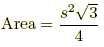# Program to Calculate Area of Equilatral Triangle

## Problem

Culculate area of equilateral triangle. And return the area with float.(2 digits after point.)

Properties of Equilateral Triangle :

``````Equilateral triangle is a triangle in which all three sides are equal .
All angles are of measure 60 degree
A three-sided regular polygon
``````

Area of this triangle :Contributed by Erkan Ercan